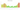# kn

## Croatian Kuna

#### how has the value of the currency changed in the last year?## USD 1 = HRK 7.1322

How much is10 US dollars worth inCroatian kunas?
At the current exchange rate, 10 US dollars is worth 71.32 Croatian kunas
How much is50 US dollars worth inCroatian kunas?
At the current exchange rate, 50 US dollars is worth 356.61 Croatian kunas
How much is100 US dollars worth inCroatian kunas?
At the current exchange rate, 100 US dollars is worth 713.22 Croatian kunas
How much is500 US dollars worth inCroatian kunas?
At the current exchange rate, 500 US dollars is worth 3,566.1 Croatian kunas
How much is2,000 US dollars worth inCroatian kunas?
At the current exchange rate, 2,000 US dollars is worth 14,264.4 Croatian kunas
How much is10 US dollars worth inCroatian kunas?
At the current exchange rate, 10 US dollars is worth 71.32 Croatian kunas
How much is50 US dollars worth inCroatian kunas?
At the current exchange rate, 50 US dollars is worth 356.61 Croatian kunas
How much is100 US dollars worth inCroatian kunas?
At the current exchange rate, 100 US dollars is worth 713.22 Croatian kunas
How much is500 US dollars worth inCroatian kunas?
At the current exchange rate, 500 US dollars is worth 3,566.1 Croatian kunas
How much is2,000 US dollars worth inCroatian kunas?
At the current exchange rate, 2,000 US dollars is worth 14,264.4 Croatian kunas